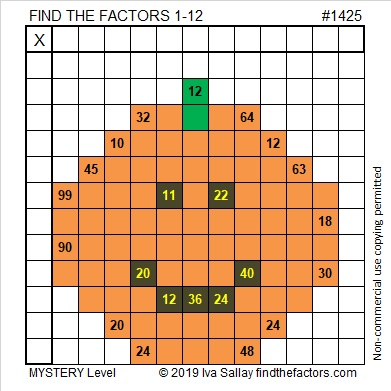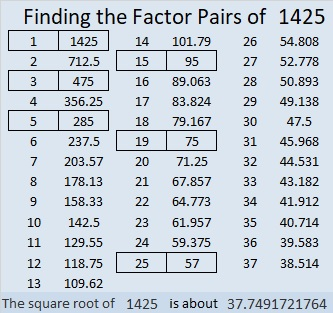# 1425 Jack O’Lantern

Most of my puzzles don’t have as many clues as this Jack O’lantern puzzle has. Those extra clues could make it easier to solve. On the other hand, some of the clues might still be tricky.Print the puzzles or type the solution in this excel file: 12 Factors 1419-1429

Here are some facts about the puzzle number, 1425:

• 1425 is a composite number.
• Prime factorization: 1425 = 3 × 5 × 5 × 19, which can be written 1425 = 3 × 5² × 19.
• 1425 has at least one exponent greater than 1 in its prime factorization so √1425 can be simplified. Taking the factor pair from the factor pair table below with the largest square number factor, we get √1425 = (√25)(√57) = 5√57.
• The exponents in the prime factorization are 1, 2, and 1. Adding one to each exponent and multiplying we get (1 + 1)(2 + 1)(1 + 1) = 2 × 3 × 2 = 12. Therefore 1425 has exactly 12 factors.
• The factors of 1425 are outlined with their factor pair partners in the graphic below.1425 is the hypotenuse of TWO Pythagorean triples:
399-1368-1425 which is (7-24-25) times 57
855-1140-1425 which is (3-4-5) times 285